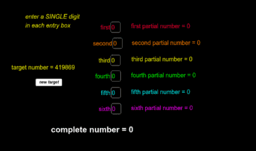# Working backwards

The applets in this collection offer opportunities to explore synthetic rather than analytic approaches to problems in arithmetic, algebra and geometry. In the first chapter we look at addition and multiplication computation problems through the lens of the inverse operations. For example, what two numbers, when added, will yield a sum between two given numbers? We also look at prime factorization by asking the user to compose numbers by multiplying primes rather than to decompose them by finding prime factors. In the second chapter we explore building polygons by linking segments to one another. For example, give three line segments that intersect at a point that subdivides each line segment in the ratio of 2:1 can one build a triangle for which the given line segments are the medians? Several of the applets allows the user to explore under what circumstances breaking a stick into n pieces the resulting pieces can be assembled into an n-sided polygon. In the third chapter we explore the building of functions – from concatenating operations and transformations on the constant and the identity functions, or by using attributes such as locations of sign changes of a function and its rate of change. Working backwards is not always possible - as the applet on non-invertable operations makes clear. In the next chapter users can explore three simple differential equations - f(x)=1/f'(x), f(x)/f'(x)=1 and f(x)+f'(x)=1. In the final two chapters we explore the UNsolving of equations and inequalities– i.e., the generating of the equivalence class of equations from the given solution set - for example, find the set of all quadratic inequalities whose solution set is -2 < x < 3. This is a collection of applets drawn from the archive website mathMINDhabits [sites.google.com/site/mathmindhabits] that is designed for teachers of mathematics who want to deepen their understanding of the mathematics they teach and that their students are expected to learn.